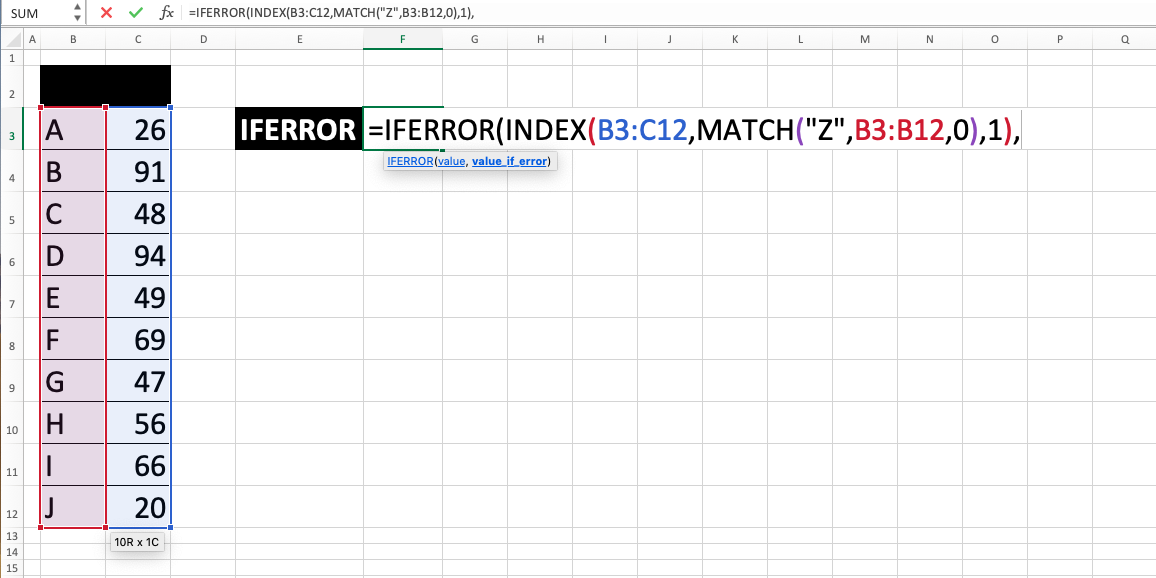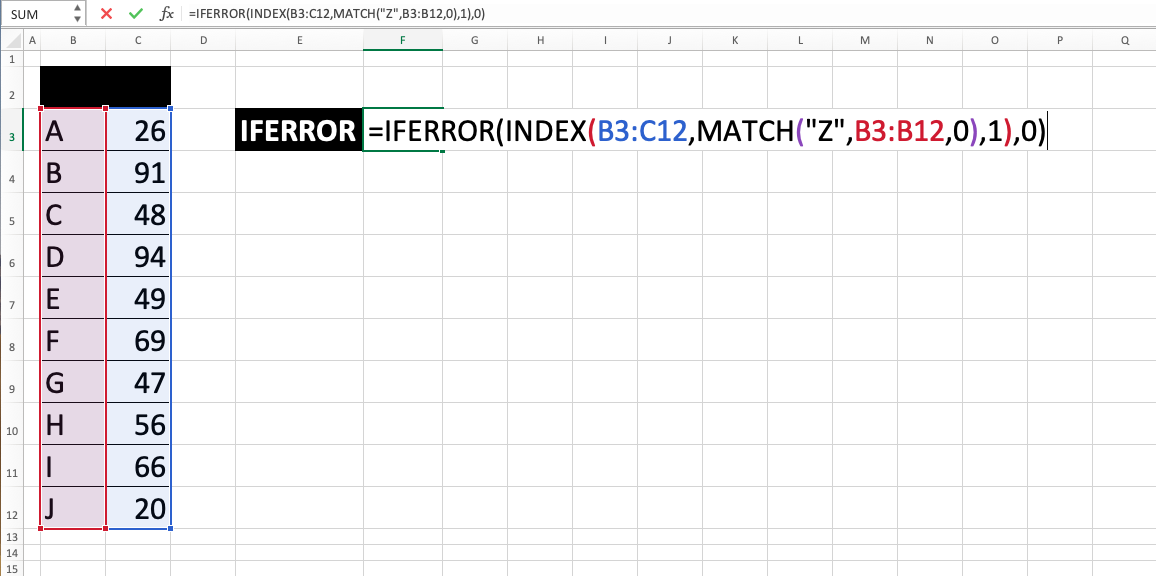IFERROR in Excel - Compute Expert

# IFERROR in Excel

In this tutorial, you will learn the details of IFERROR excel usage. In brief, the function of IFERROR in excel is useful in giving us alternative output value if something that we input in a cell has a value or produces an error.

## Why We Need to Learn IFERROR Excel?

While doing data processing in a spreadsheet, especially when we write a formula in a cell, sometimes there is an error value as a result of the processing that is unexpected by us.

This kind of thing can disturb our data processing heavily because, as a result, we cannot get the outcome as we want from the process. It can also make other cells that refer to the outcome give an error value too. Besides that, that kind of value can also make the spreadsheet interface bad when we want to show our data processing results to other people (it looks unprofessional because it is as if there are many mistakes in the data processing that we do).

As a situation example illustration, imagine if we are doing data processing where there is a need to look for a particular data from a table reference in the spreadsheet. In doing the data search, usually, we use formulas like VLOOKUP, HLOOKUP or INDEX MATCH combination. But sometimes, and of course this is not planned before, the data that we look for can’t be found in the reference table so the formula will result in an error. When the result is referenced as an input for another formula, the one that uses the reference input will give an error too. From here, we can see a negative impact cycle on the whole data processing that we do in the spreadsheet.

Potentially, this can screw up our data analysis severely.

To solve the problem, it will be great if there is a function that can be used to anticipate this kind of value that can be produced from a technical mistake. For this matter, you can use the formula of IFERROR in excel.

IFERROR excel can help by giving alternative value automatically if your input in the formula produces an error (Because of this, usually its input is the writing of another formula). It is very useful if you often use formulas, which we, as excel users, often use those things, in your data processing on this software.

## What is IFERROR in Excel?

IFERROR in excel is a formula with the usage objective to give alternative value if your input in this IFERROR excel formula has or produces an error value. It is very often used for anticipation when we don’t know whether a formula that is inputted in a cell will correctly produce a value that is needed or, instead, produce an error.

A bit explanation about the inputs in it as follows:

=IFERROR(value, value_if_error)

Note:
• value = data or formula that you want to test whether the value is error or not
• value_if_error = alternative value to be produced if it is true that the data or formula is an error

## How to Use IFERROR Excel?

The following will explain the details of how to write IFERROR in excel. The input needs of IFERROR excel formula are the data or formula where there is an anticipation of error in its value and what is the alternative value that you want to produce if it is true that the data or formula will later produce an error.

This function will just give the data or formula as the result if there is no error. But, in another time, it will produce the alternative value if the data or formula actually has an error value.

## Using IFERROR in Excel

1. Type equal sign ( = ) in the cell where you want to put the result in2. Type IFERROR (can be with large and small letters) and open bracket sign after =3. Type data or formula or cell coordinate where the data or formula that you want to anticipate an error value from is, then type a comma sign ( , )4. Type the alternative value or cell coordinate where the value that will be produced if your previously inputted data input or formula has an error value is5. Type close bracket sign6. Press Enter
7. The process is done!

The result with IFERROR excelThe result without IFERROR excel## Exercise

After you have learned the detailed tutorial of IFERROR excel function above, you can practice your understanding by doing the exercise below!

### Questions

Anticipate error on each answer using IFERROR in excel! Use the alternative value of “No data” for each of the anticipation!
1. Find the price per dozen from the left table for the products which are planned to be sold in region 1! Using VLOOKUP or INDEX MATCH to find it. Learn the tutorial in other parts of Compute Expert if you still don’t know how to use one of them!
2. Find the price per unit also by calculating it from the price per dozen for all products in region 1!
3. Find the price per dozen after discount!

• If you want to specify the anticipation just for #NA (Not Available, happens when something that you want to get is not available) type of error, then you better use IFNA. The way how to use IFNA is the same with IFERROR in excel.
• Error identification without using IF function can be done by using ISERROR formula

Get updated excel info from Compute Expert by registering your email. It's free!